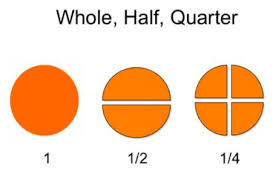# FRACTION

HELLO CHILDREN, WELCOME BACK TO CLASS

SUBTOPIC: ½ AND ¼ OF A GIVEN COLLECTION OF CONCRETE OBJECTS

In primary 1 we learnt that fraction is a part of a whole.

ACTIVITY

• Take one A4 paper and divide it into two equal parts.
• Also take an orange and divide it into two equal parts.

Each piece of the divided objects is referred to as HALF which stands for ½ in maths. So, half (½) is one of two equal parts

ACTIVITY

• Take each half of the object divided above and divide into two equal parts such that you have four parts of the original object. Each of these four parts is called QUARTER which stands for ¼ in maths. So, quarter (¼) is one part out of four equal parts.So If i have four cups of tea—- ☕☕☕☕ and I divide them into two equal parts  ☕☕        ☕☕

I will get 2 each, so ½ of 4 is 2.

In the same way, 8 flowers divided into four     🌷🌷           🌷🌷         🌷🌷       🌷🌷 will give us 2 so ¼ of 8 is 2

ACTIVITY

• Find the following
1. i) ½ of 8 eggs
2. ii) ½ of 10 oranges

iii) ¼ of 12 pencils

1. iv) ¼ of 16 balls
2. v) ¼ of 14 knives
3. vi) ½ of 18 wrist watches.

Your Opinion Matters! Quickly tell us how to improve your Learning Experience HERE